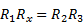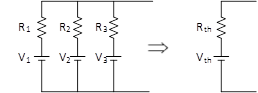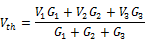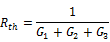Contents

POWER

POWER IN AC CIRCUITS

Average, MAX and min PoweR

Complex Power

Power factor correction

Trigonometric identites Frequently used in complex power calculations

INDUCTORS

TRANSFORMERS

DOT convention

Bridge Circuits

Millman’s Theorem

# POWER

## POWER IN AC CIRCUITS

### Average, MAX and min PoweR

 Signal Instantaneous Power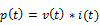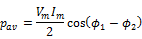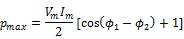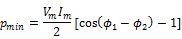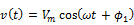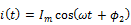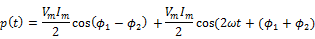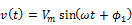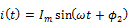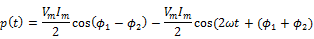Average , Maximum and Minimum power in terms of power factor (PF)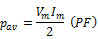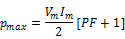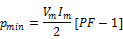### Complex Power

 Complex Power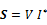1.       Apparent Power is the magnitude of the complex power.   2.       Average/Real Power and Reactive Power is the real part and imaginary part of the complex power respectively.   3.       Unit of reactive power is VARS Reactive Power (VARS)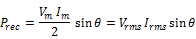Average Power/Real Power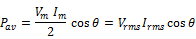Complex Power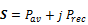1.       Apparent Power =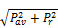2.       The unit of apparent power is VA   3.       Apparent power is the magnitude of the complex power.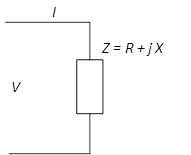1.       Complex Power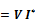2.       Real Power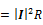3.       Reactive Power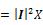4.       Apparent Power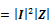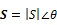### Power factor correction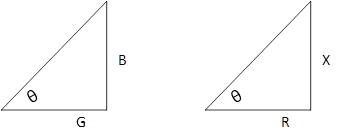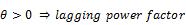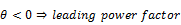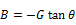(Lagging power factor)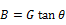(Leading power factor)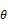is the impedance angle, or power factor angle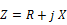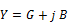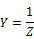### Trigonometric identites Frequently used in complex power calculations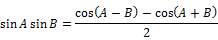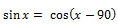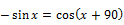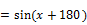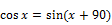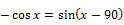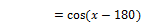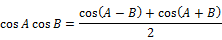Average value of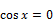Average value of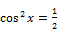Inductance of a coil with N turns, cross section area A, length L and core permeability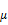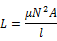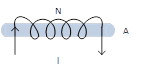Voltage induced across an inductor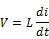Voltage induced across a coil (w.r.t flux linkage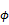)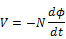Flux linkageand current relation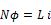Energy stored in an inductor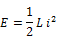Magnetic equivalent of electric current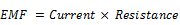Source of EMF is battery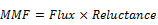Source of MMF is current coil

## DOT convention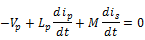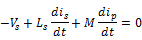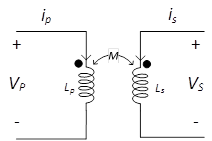A current entering the dotted terminal of one coil produces voltage with a positive voltage reference at the dotted terminal of the second coil.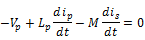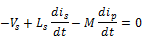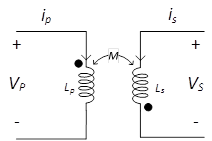A current entering the dotted terminal of one coil produces voltage with a positive voltage reference at the dotted terminal of the second coil. Voltages and currents in terms of phasors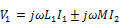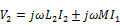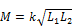Coefficient of mutual coupling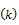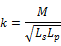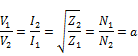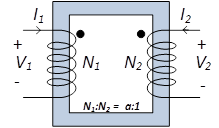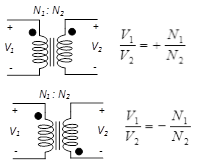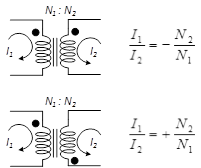Dot convention and current / voltage polarity reference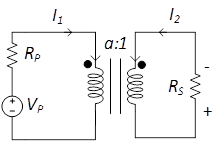Reflection of secondary into primary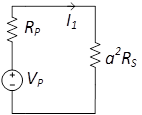Reflection of primary into secondary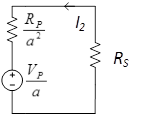Current and flux direction using right hand rule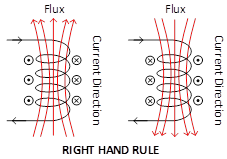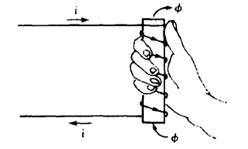LENZ’S LAW   The direction of the induced voltage( in other words the current direction)will be such that it will oppose the change in the flux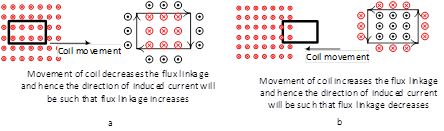General Transformer Equation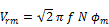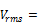primary or secondary RMS Voltage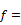Frequency of RMS Voltage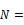turns of primary or secondary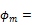Peak value of flux Impedance Matching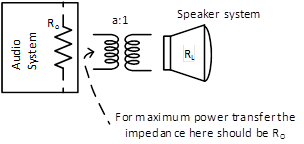If the transformer is acting as an impedance matching device than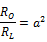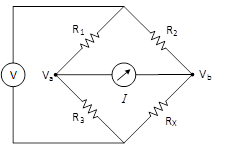Bridge circuit can be used to determine the value of an unknown resistance.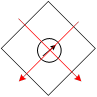The bridge is balanced if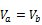or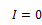.   When the bridge is balanced then